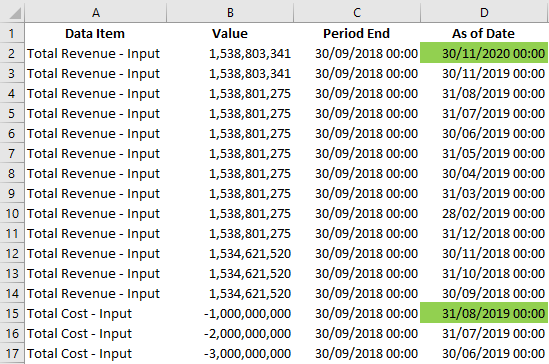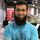cancel
Showing results for
Did you mean:Frequent Visitor

## Max / Last date on a filtered range

Hi All,

Hopefully an easy question.

I have a data set where the value of a period is stored every subsequent period, where the period value is the same but the 'as at period' is monthly. The value to be referenced is the one represented in the latest 'as at period'.

In the below table I only want to bring back the two values highlighted in green.1 ACCEPTED SOLUTIONFrequent Visitor

I solved my issue.

I created a calculated column which concatenated the elements which would impact my filter, then using that column as a variable I filtered my table using MAXX on the As At date.

So far the numbers reconcile.

2 REPLIES 2Frequent Visitor

I solved my issue.

I created a calculated column which concatenated the elements which would impact my filter, then using that column as a variable I filtered my table using MAXX on the As At date.

So far the numbers reconcile.Super User

I am sure there is a more efficient way to to do this so I will let the experts improve on my answer (note this is a calculated table you need to create)

``````AsAtPeriod =
SUMMARIZE (
'Table',
'Table'[Data Item],
"MAXDATE",
CALCULATE (
MAX ( 'Table'[As of Date] ),
ALLEXCEPT ( 'Table', 'Table'[Data Item] )
),
"VAL",
CALCULATE (
SUM ( 'Table'[Value] ),
FILTER (
'Table',
'Table'[As of Date]
= CALCULATE (
MAX ( 'Table'[As of Date] ),
ALLEXCEPT ( 'Table', 'Table'[Data Item] )
)
)
)
)``````Regards,

Moiz

If this post helps, please "Accept" it as Solution to help other members find it.

Thanks,

Moiz
Was I able to answer your question? Mark my post as a solution to help others. Kudos if you liked the solution.
Proud to be a Super User!